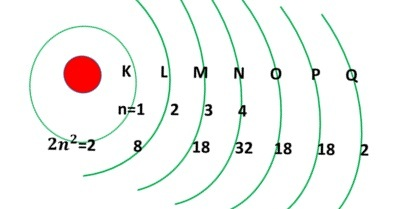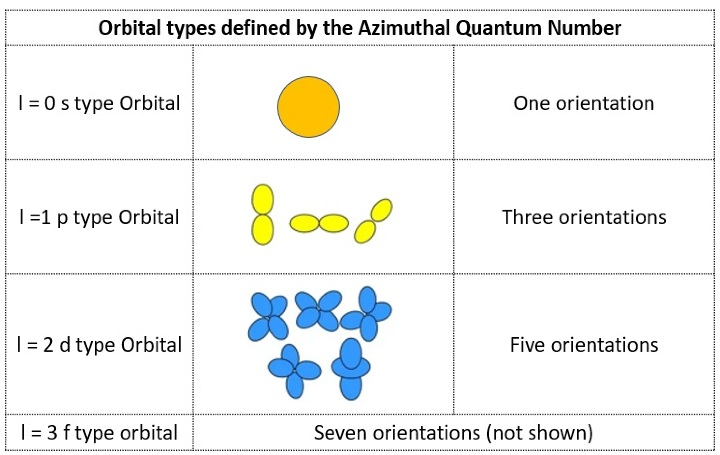# Azimuthal Quantum Number - Definition, subsidiary, and FAQs

## Introduction

Azimuthal quantum number and their study are important to understand the quantum nature of matter. Atoms are a basic unit of a material. According to the Bohr atom model atoms contain a small central core called a nucleus which is full of positive charges. Electrons are round about the nucleus in a particular circle named orbits. The circular path is maintained by the centripetal force derived from the electrostatic force between the electrons and the nucleus. Different orbits have different energy. As it fails to explain Heisenberg’s uncertainty principle and particle’s dual nature a new branch called quantum mechanics was formed. It was developed by Erwin Schrodinger. He developed this in accordance with the wave nature of particles like electrons. The main concepts of quantum mechanics are quantization of energy, the duality nature of particles, and the uncertainty principle.

## What are Quantum Numbers?

Quantum numbers are used to locate the electrons. It gives a description of the energy levels of the atom. Different orbits have different sizes, shapes, and orientations. Quantum numbers represent the different characteristics of the orbits of an atom. That means quantum numbers give complete information about electrons like size, shape, location, and orientation in space. There are four quantum numbers to explain the status of electrons. They are

• Principal quantum number (n).

• Magnetic quantum number (ml).

• Azimuthal quantum number (l).

• Spin quantum number (ms).

## What is the Principal Quantum Number?

This number provides information about the energy orbits of an electron. How far the electrons are located from the nucleus is indicated by the principal quantum number. It is denoted by the symbol n. It takes the value of positive integers from 1 to infinite (i.e) n=1,2,3................

According to the value of n, the shells are indicated by,K,L,M,N,O, etc.

Occupation of electrons for each orbital is defined by $\mathrm{2n^2}$

If n=1, then the K-orbital has two electrons.

If n=2 then L-orbitalhas 8 electrons and so on.If the electron from the lower energy level exited to the higher energy level, then the value of n increased and it is called absorption. If the electron from the upper state is jumped to the lower state, then the value of n decreases which is called emission. During absorption electrons absorb energy and during emission electrons emit their energy.

The principal quantum number also gives an equation to find the energy of a hydrogen atom as,

$$\mathrm{En =-\frac{2\pi^2 me^4 Z^2}{n^2 h^2}}$$

The energy values of the orbits are increased from K to Q.

## Definition: Azimuthal Quantum Number

This number gives the information about the configuration of the orbital by finding its orbital angular momentum. In a multiple electron atom, the energy path of electrons is different and so their angular momentum will differ, (i.e) there are subshells in the multi-electron atom. This number is denoted by l. The azimuthal quantum number is decided by the principal quantum number. It gives details about the shape, energy, and angular momentum of the subshells. Azimuthal quantum numbers l carries the value 0 to (n-1).

They are denoted by s, p, d, f ….Let us take n=1, it is a K-shell.

The Azimuthal values are l=0. It has only one subshell. It is 1s.

If n=2, it is a L-shell.

The Azimuthal values are l=0,1. It has two subshells. They are 2s,2p.

If n=3, it is a M-shell.

The Azimuthal values are l=0,1.2. It has three subshells. They are 3s,3p,3d.

Principal quantum number(n)ShellAzimuthal quantum number(l)No of SubshellSubshells
1K011s
2L0,122s, 2p
3M0,1,233s, 3p, 3d
4N0,1,2,344s, 4p, 4d, 4f

Table-1: Filling Principal quantum number and Azimuthal Quantum number

Thus, the number of subshells and principal quantum numbers are the same.

## History of Azimuthal Quantum Number

Bohr made use of the concept of quantization and proposes his theory. According to his theory, the electron revolves around the nucleus without losing energy. It gains energy when electrons move from a lower energy state to a higher and it loses energy when electrons move from a higher state to a lower one. He denoted the energy levels by quantum numbers, n=1,2,3...........as K,L,m, etc. But it failed to explain the electric and magnetic field effect of atoms and also his concepts became applicable only to smaller atoms. It also failed to explain the additional behaviour of electrons. Three more quantum numbers were added in addition to the principal quantum number to describe the behaviour of a multi-electron atom.

The azimuthal quantum number was taken over from the Bohr atom model. The lowest energy level has an angular momentum of zero. That is, it was considered as one dimension. In three dimensions it oscillates like a pendulum in a circular path.

## Conclusion

Atom consists of a nucleus at the center and is surrounded by electrons. Quantum numbers are numerically used to locate the electron's position. All four quantum numbers give information about the shape, size, and orientation of electrons. Principal quantum number and Azimuthal quantum number were discussed here.

## FAQs

Q1. What is Pauli's exclusion principle?

Ans. Pauli’s exclusion principle is the theory of quantum mechanics. According to this theory, no two fermions can be in the same state. For electrons, two electrons cannot be in the same quantum state.

Particles are named fermions if they obey this principle. ex: electrons, atoms, neutrons.

Particles are named bosons if they do not obey this principle. ex: photons.

Q2. State Hund’s Rule.

Ans. This rule explains the distribution of electrons in the orbitals. It states that first each sub-orbitals of the orbital are occupied by a single electron. After that only, the pairing of electrons will happen.

Q3. What is the importance of quantum numbers?

Ans. Quantum numbers describe the position of electrons in space. Schrodinger wave equation in accordance with the four quantum numbers gives complete information on the characteristics of electrons. As per Pauli’s exclusion principle, two electrons cannot be in the four quantum levels. These quantum number talks about the energy levels, sub-shells of the atoms, and orientation of electrons. The spin quantum number shows the spinning direction of electrons.

Q4. What is Heisenberg’s Uncertainty principle?

Ans. Heisenberg’s Uncertainty principle describes that it is impossible to find both the position and momentum of the electron simultaneously at the same time.

Q5. What is an Atomic Orbital?

Ans. A mathematical quantity that represents the location of an electron and its wave nature is referred to as atomic orbital.

Updated on: 18-Apr-2023

117 Views